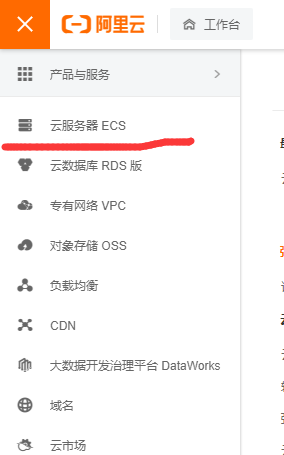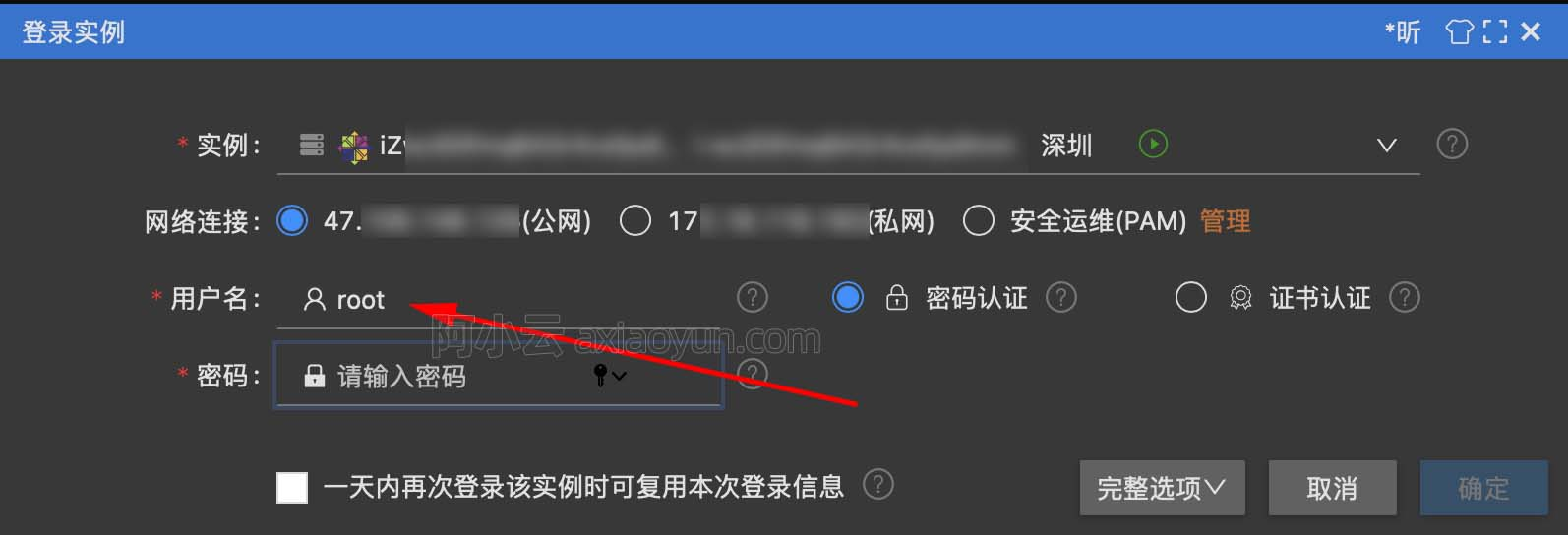MonetDB 1.6 billion(384GB) JOIN 2.4 billion(576GB) 60 columns-random-data wide-table

+关注继续查看

MonetDB vs PostgreSQL 2, width table with random data
MonetDB bulk load performance & fixed length type performance & JOIN performance
PostgreSQL fixed length wide-table VS MonetDB
PostgreSQL cstore_fdw column-ORI table VS MonetDB (with fixed-length width table)

sql>select count(*) from bt1;
+------------+
| L1         |
+============+
| 2400000000 |
+------------+
1 tuple (8.203ms)
sql>select count(*) from bt2;
+------------+
| L1         |
+============+
| 1600000000 |
+------------+
1 tuple (9.380ms)

sql>select "schema","table",sum(columnsize)/1024/1024/1024.0||'GB' from storage group by "schema","table" order by sum(columnsize) desc;
+--------+-------------------+-----------+
| schema | table             | L2        |
+========+===================+===========+
| sys    | bt1               | 536.441GB |
| sys    | bt2               | 357.626GB |
| sys    | bt5               | 135.599GB |
| sys    | bt4               | 84.750GB  |
| sys    | bt3               | 50.849GB  |

and和or的效率差别比较大. or的列越多, 查询越慢.
and查询, 不够多少个条件, 性能都比较平稳, 因为2个条件就已经可以毙掉所有的结果了.
and和or的查询一般用在标签场景, 例如按条件搜索商品等.
sql>select count(*) from bt1 where c1=1;
+------+
| L1   |
+======+
|   48 |
+------+
1 tuple (12.9s)
sql>select count(*) from bt1 where c1=1;
+------+
| L1   |
+======+
|   48 |
+------+
1 tuple (5.5s) -- 第二次不需要从磁盘读取.
sql>select count(*) from bt1 where c1=1 and c2=1;
+------+
| L1   |
+======+
|    0 |
+------+
1 tuple (12.7s)  -- c2列需要从磁盘读取
sql>select count(*) from bt1 where c1=1 and c2=1 and c3=1;
+------+
| L1   |
+======+
|    0 |
+------+
1 tuple (13.3s)  -- 不需要把所有的数据载入内存, 因为只需要C1+C2个列的数据就可以得出所有都是false的结论了, 多余的列都不需要判断.
sql>select count(*) from bt1 where c1=1 and c2=1 and c3=1 and c4=1;
+------+
| L1   |
+======+
|    0 |
+------+
1 tuple (12.8s)
sql>select count(*) from bt1 where c1=1 and c2=1 and c3=1 and c4=1 and c5=1;
+------+
| L1   |
+======+
|    0 |
+------+
1 tuple (13.7s)
sql>select count(*) from bt1 where c1=1 and c2=1 and c3=1 and c4=1 and c5=1 and c6=1;
+------+
| L1   |
+======+
|    0 |
+------+
1 tuple (15.3s)
sql>select count(*) from bt1 where c1=1 and c2=1 and c3=1 and c4=1 and c5=1 and c6=1 and c7=1;
+------+
| L1   |
+======+
|    0 |
+------+
1 tuple (5.4s)
sql>select count(*) from bt1 where c1=1 and c2=1 and c3=1 and c4=1 and c5=1 and c6=1 and c7=1 and c8=1;
+------+
| L1   |
+======+
|    0 |
+------+
1 tuple (16.8s)
sql>select count(*) from bt1 where c1=1 and c2=1 and c3=1 and c4=1 and c5=1 and c6=1 and c7=1 and c8=1 and c9=1;
+------+
| L1   |
+======+
|    0 |
+------+
1 tuple (17.7s)
sql>select count(*) from bt1 where c1=1 and c2=1 and c3=1 and c4=1 and c5=1 and c6=1 and c7=1 and c8=1 and c9=1 and c10=1;
+------+
| L1   |
+======+
|    0 |
+------+
1 tuple (14.2s)
sql>select count(*) from bt1 where c1=1 and c2=1 and c3=1 and c4=1 and c5=1 and c6=1 and c7=1 and c8=1 and c9=1 and c10=1 and c11=1;
+------+
| L1   |
+======+
|    0 |
+------+
1 tuple (15.1s)
sql>select count(*) from bt1 where c1=1 and c2=1 and c3=1 and c4=1 and c5=1 and c6=1 and c7=1 and c8=1 and c9=1 and c10=1 and c11=1 and c12=1;
+------+
| L1   |
+======+
|    0 |
+------+
1 tuple (17.4s)
sql>select count(*) from bt1 where c1=1 and c2=1 and c3=1 and c4=1 and c5=1 and c6=1 and c7=1 and c8=1 and c9=1 and c10=1 and c11=1 and c12=1 and c13=1;
+------+
| L1   |
+======+
|    0 |
+------+
1 tuple (18.0s)
sql>select count(*) from bt1 where c1=1 and c2=1 and c3=1 and c4=1 and c5=1 and c6=1 and c7=1 and c8=1 and c9=1 and c10=1 and c11=1 and c12=1 and c13=1 and c14=1;
+------+
| L1   |
+======+
|    0 |
+------+
1 tuple (23.1s)
sql>select count(*) from bt1 where c1=1 and c2=1 and c3=1 and c4=1 and c5=1 and c6=1 and c7=1 and c8=1 and c9=1 and c10=1 and c11=1 and c12=1 and c13=1 and c14=1 and c15=1;
+------+
| L1   |
+======+
|    0 |
+------+
1 tuple (20.4s)
sql>select count(*) from bt1 where c1=1 and c2=1 and c3=1 and c4=1 and c5=1 and c6=1 and c7=1 and c8=1 and c9=1 and c10=1 and c11=1 and c12=1 and c13=1 and c14=1 and c15=1 and c16=1;
+------+
| L1   |
+======+
|    0 |
+------+
1 tuple (20.2s)
sql>select count(*) from bt1 where c1=1 and c2=1 and c3=1 and c4=1 and c5=1 and c6=1 and c7=1 and c8=1 and c9=1 and c10=1 and c11=1 and c12=1 and c13=1 and c14=1 and c15=1 and c16=1 and c17=1 and c18=1 and c19=1 and c20=1 and c21=1 and c22=1 and c23=1 and c24=1 and c25=1 and c26=1 and c27=1 and c28=1 and c29=1 and c30=1 and c31=1 and c32=1 and c33=1 and c34=1 and c35=1 and c36=1 and c37=1 and c38=1 and c39=1 and c40=1;
+------+
| L1   |
+======+
|    0 |
+------+
1 tuple (15.2s)

or的查询因为每列都要判断, 所以在本例and得出为0的情况下, 判断的字段越多, and和or的性能差别就越大.
sql>select count(*) from bt1 where c1=1 or c2=1;
+------+
| L1   |
+======+
|  240 |
+------+
1 tuple (49.6s)
sql>select count(*) from bt1 where c1=1 or c2=1;
+------+
| L1   |
+======+
|  240 |
+------+
1 tuple (17.3s)
sql>select count(*) from bt1 where c1=1 or c2=1 or c3=1 or c4=1 or c5=1 or c6=1 or c7=1 or c8=1 or c9=1 or c10=1 or c11=1 or c12=1 or c13=1 or c14=1 or c15=1 or c16=1 or c17=1 or c18=1 or c19=1 or c20=1 or c21=1 or c22=1 or c23=1 or c24=1 or c25=1 or c26=1 or c27=1 or c28=1 or c29=1 or c30=1 or c31=1 or c32=1 or c33=1 or c34=1 or c35=1 or c36=1 or c37=1 or c38=1 or c39=1 or c40=1;
+------+
| L1   |
+======+
| 2160 |
+------+
1 tuple (33m 5s)

sql>select count(bt1.c1) from bt1 join bt2 on (bt1.c1=bt2.c2 and bt1.c2=bt2.c3 and bt1.c3=bt2.c4 and bt1.c5<10000);
+------+
| L1   |
+======+
|    0 |
+------+
1 tuple (15m 27s)

sql>\d bt3
CREATE TABLE "sys"."bt3" (
"id" INTEGER
);
sql>
sql>\d bt4
CREATE TABLE "sys"."bt4" (
"id" INTEGER
);
sql>
sql>\d bt5
CREATE TABLE "sys"."bt5" (
"id" INTEGER
);

sql>select * from storage where "table"='bt5';   -- 147GB
+--------+-------+--------+------+----------+-------------+-----------+--------------+----------+---------+--------+
| schema | table | column | type | location | count       | typewidth | columnsize   | heapsize | indices | sorted |
+========+=======+========+======+==========+=============+===========+==============+==========+=========+========+
| sys    | bt5   | id     | int  | 22/2242  | 36400000000 |         4 | 145600000000 |        0 |       0 | false  |
+--------+-------+--------+------+----------+-------------+-----------+--------------+----------+---------+--------+
1 tuple (12.695ms)
sql>select * from storage where "table"='bt4';   -- 91GB
+--------+-------+--------+------+----------+-------------+-----------+-------------+----------+---------+--------+
| schema | table | column | type | location | count       | typewidth | columnsize  | heapsize | indices | sorted |
+========+=======+========+======+==========+=============+===========+=============+==========+=========+========+
| sys    | bt4   | id     | int  | 41/4115  | 22750000000 |         4 | 91000000000 |        0 |       0 | false  |
+--------+-------+--------+------+----------+-------------+-----------+-------------+----------+---------+--------+
1 tuple (5.738ms)
sql>select * from storage where "table"='bt3';   -- 56GB
+--------+-------+--------+------+-------------+-------------+-----------+-------------+----------+---------+--------+
| schema | table | column | type | location    | count       | typewidth | columnsize  | heapsize | indices | sorted |
+========+=======+========+======+=============+=============+===========+=============+==========+=========+========+
| sys    | bt3   | id     | int  | 02/25/22500 | 13650000000 |         4 | 54600000000 |        0 |       0 | false  |
+--------+-------+--------+------+-------------+-------------+-----------+-------------+----------+---------+--------+
1 tuple (6.381ms)

sql>select count(*) from bt3;
+-------------+
| L1          |
+=============+
| 13650000000 |
+-------------+
1 tuple (56.279ms)
sql>select count(*) from bt4;
+-------------+
| L1          |
+=============+
| 22750000000 |
+-------------+
1 tuple (52.546ms)
sql>select count(*) from bt5;
+-------------+
| L1          |
+=============+
| 36400000000 |
+-------------+
1 tuple (55.480ms)

364亿插入性能 :
sql>insert into bt5 select * from bt4 union all select * from bt3;
36400000000 affected row (17m 54s)

sql>select count(*) from bt3 where id=1;
+------+
| L1   |
+======+
|  273 |
+------+
1 tuple (50.3s)
sql>select count(*) from bt5 where id=1;
+------+
| L1   |
+======+
|  728 |
+------+
1 tuple (5m 47s)

sql>select count(bt5.id) from bt5 join bt1 on (bt5.id=bt1.c2 and bt5.id=bt1.c3 and bt5.id<100) ;
+------+
| L1   |
+======+
|    0 |
+------+
1 tuple (43m 25s)

[参考]
1. MonetDB vs PostgreSQL 2, width table with random data
2. MonetDB bulk load performance & fixed length type performance & JOIN performance
3. PostgreSQL fixed length wide-table VS MonetDB
4. PostgreSQL cstore_fdw column-ORI table VS MonetDB (with fixed-length width table)10061 026784 02508 0Xshell使用SSH远程登录阿里云ECS服务器CentOS7
13107 012070 011817 0windows server 2008阿里云ECS服务器安全设置

9157 04654 04498 07360 0+关注

2153

245

《2021云上架构与运维峰会演讲合集》

《零基础CSS入门教程》

《零基础HTML入门教程》